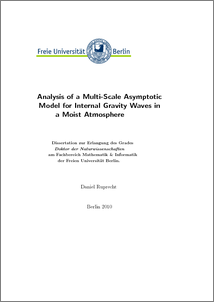Repository: Freie Universität Berlin, Math Department

# Analysis of a Multi-Scale Asymptotic Model for Internal Gravity Waves in a Moist Atmosphere

Ruprecht, D. (2010) Analysis of a Multi-Scale Asymptotic Model for Internal Gravity Waves in a Moist Atmosphere. PhD thesis, Freie Universität Berlin.Preview

3MB

## Abstract

The thesis presents the analysis of a reduced model for modulation of internal gravity waves by deep convective clouds. The starting point for the derivation are conservation laws for mass, momentum and energy coupled with a bulk micro-physics model describing the evolution of mixing ratios of water vapor, cloud water and rain water. A reduced model for the identified scales of the regime is derived, using multi-scale asymptotics. The closure of the model employs conditional averaging over the horizontal scale of the convective clouds. The resulting reduced model is an extension of the anelastic equations, linearized around a constant background state, which are well-known from meteorology. The closure of the model is achieved purely by analytical means and involves no additional physically motivated assumptions. The essential new parameter arising from the coupling to a micro-physics model is the area fraction of saturated regions on the horizontal scale of the convective clouds. It turns out that this parameter is constant on the employed short timescale. Hence the clouds constitute a constant background, modulating the characteristics of propagation of internal waves. The model is then investigated by analytical as well as numerical means. Important results are, among others, that in the model moisture (i) inhibits propagation of internal waves by reducing the modulus of the group velocity, (ii) reduces the angle between the propagation direction of a wave-packet and the horizontal, (iii) causes critical layers and (iv) introduces a maximum horizontal wavelength beyond which waves are no longer propagating but become evanescent. The investigated examples of orographically generated gravity waves also feature a significant reduction of vertical momentum flux by moisture. The model is extended by assuming systematically small under-saturation, that is saturation at leading order. The closure is similar to the original case but requires additional assumptions. The saturated area fraction in the obtained model is no longer constant but now depends nonlinearly on vertical displacement and thus on vertical velocity.

Item Type: Thesis (PhD) Mathematical and Computer Sciences > Mathematics > Applied Mathematics Department of Mathematics and Computer Science > Institute of Mathematics > Geophysical Fluid Dynamics Group 917 Ulrike Eickers 26 Jul 2010 12:32 03 Mar 2017 14:40

Repository Staff Only: item control page